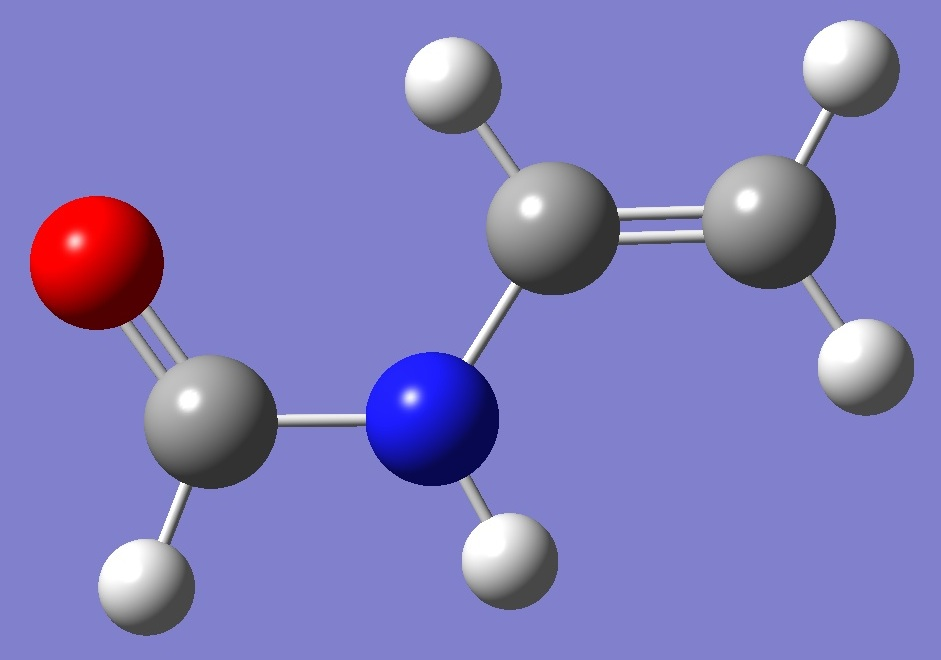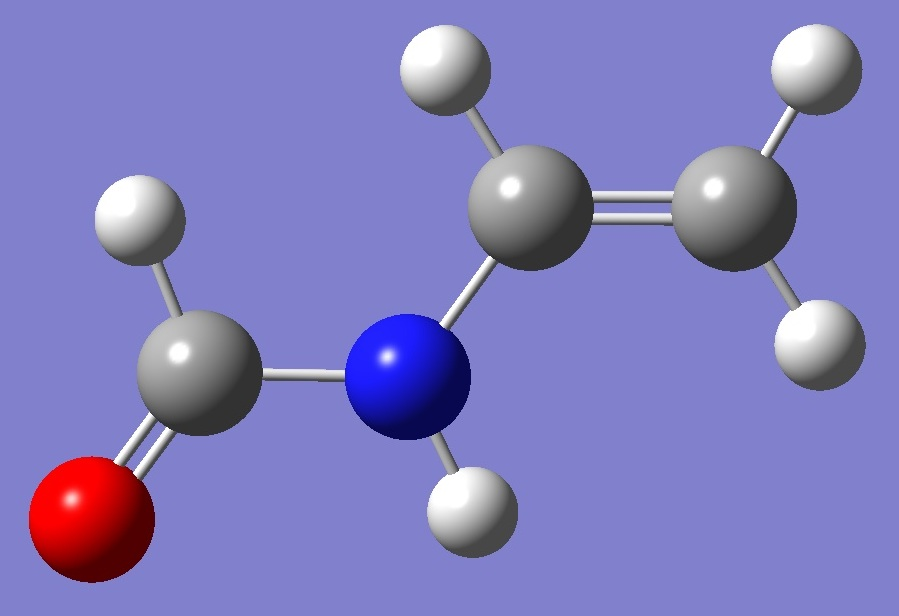CH2=CH-NH-C(=O)H

Nitrogen

in N-Vinylformamide

The microwave spectrum of N-vinylformamide was first investigated by Møllendal and Samdal , which authors observed and established the planarity of the trans and cis conformers shown below.  Subsequently, 14N nqcc's were determined by Kannengießer, et al. .

trans CH2=CH-NH-C(=O)H

cis CH2=CH-NH-C(=O)HAt MP2/6-311++G(d,p) level of theory, Etrans < Ecis by 1.8 kJ/mol.

Calculation was made of the 14N nqcc tensor on ropt molecular structures derived by MP2/6-311++G(d,p) optimization .  These are compared with the experimental nqcc's in Tables 1 and 2.  Structure parameters are given in Table 3.

In Tables 1 and 2, subscripts a,b,c refer to the principal axes of the inertia tensor; x,y,z to the principal axes of the nqcc tensor.  ETA = (Xxx - Xyy)/Xzz.

RSD are the calibration residual standard deviation of the B3PW91/6-311+G(df,pd) and B3PW91/6-311+G(d,p) models for calculation of the efg's/nqcc's.

 Table 1. 14N nqcc's in trans CH2=CH-NH-C(=O)H (MHz).  Calculation was made on the ropt structure with (1) B3PW91/6-311+G(df,pd) and (2) B3PW91/6-311+G(d,p) models. Calc (1) Calc (2) Expt  Xaa 1.622 1.714 1.70574(90) Xbb 1.893 1.955 1.9358(11) Xcc - 3.515 - 3.669 - 3.6416(11) |Xab| 0.060 0.070 RMS 0.091 (3.7 %) 0.020 (0.82 %) RSD 0.030 (1.3 %) 0.086 (3.8 %) Xxx 1.610 1.695 Xyy 1.906 1.974 Xzz - 3.515 * - 3.669 * ETA 0.0842 0.0759

* The z-principal axis is perpendicular to the plane of symmetry of the molecule.

 Table 2. 14N nqcc's in cis CH2=CH-NH-C(=O)H (MHz).  Calculation was made on the ropt structure with (1) B3PW91/6-311+G(df,pd) and (2) B3PW91/6-311+G(d,p) models. Calc (1) Calc (2) Expt  Xaa 1.752 1.847 1.8520(14) Xbb 1.701 1.759 1.7876(20) Xcc - 3.454 - 3.606 - 3.6396(20) |Xab| 0.151 0.151 RMS 0.131 (5.4 %) 0.026 (1.1 %) RSD 0.030 (1.3 %) 0.086 (3.8 %) Xxx 1.573 1.645 Xyy 1.880 1.961 Xzz - 3.454 * - 3.606 * ETA 0.0890 0.0875

* The z-principal axis is perpendicular to the plane of symmetry of the molecule.

 Table 3.  N-Vinylformamide.  MP2/6-311++G(d,p) ropt structure parameters.  (Å and degrees). C  C,1,B1  N,1,B2,2,A1  C,3,B3,1,A2,2,D1,0  O,4,B4,3,A3,1,D2,0  H,1,B5,2,A4,3,D3,0  H,2,B6,1,A5,3,D4,0  H,2,B7,1,A6,3,D5,0  H,4,B8,3,A7,1,D6,0  H,3,B9,1,A8,2,D7,0 C  C,1,B1  N,1,B2,2,A1  C,3,B3,1,A2,2,D1,0  O,4,B4,3,A3,1,D2,0  H,1,B5,3,A4,4,D3,0  H,2,B6,1,A5,3,D4,0  H,2,B7,1,A6,3,D5,0  H,4,B8,3,A7,1,D6,0  H,3,B9,1,A8,2,D7,0 trans cis B1=1.3417365  B2=1.39929396  B3=1.3758359  B4=1.21663171  B5=1.08418612  B6=1.08567916  B7=1.08210411  B8=1.1037552  B9=1.01084055  A1=123.91982201  A2=123.22195749  A3=124.96366679  A4=122.81240583  A5=122.21166317  A6=119.61894852  A7=111.7887673  A8=119.04529762  D1=180.  D2=0.  D3=180.  D4=0.  D5=180.  D6=180.  D7=0. B1=1.34219018  B2=1.39561164  B3=1.3740195  B4=1.21619249  B5=1.0865399  B6=1.0850312  B7=1.08188585  B8=1.10427614  B9=1.01350557  A1=124.70892026  A2=124.25365678  A3=124.33190674  A4=113.86274933  A5=121.99072264  A6=119.64380536  A7=112.22720839  A8=119.54266375  D1=180.  D2=180.  D3=0.  D4=0.  D5=180.  D6=0.  D7=0.

 H.Møllendal and S.Samdal, J.Phys.Chem. A 116,12073(2012).

 R.Kannengießer, W.Stahl, H.V.L.Nguyen, and W.C.Bailey, J.Mol.Spectrosc. 317,50(2015).

Formamide N-Methylformamide
Uracil

N-Methyldiacetamide
2-Pyridone
Amides

Molecules/Nitrogen

CH2CHNHCOH.html